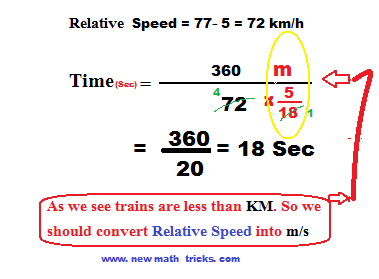# Answered question from Train's Speed Chapter

As all of you know that the competitive exam paper becoming more complicated day by day. So we have to earn more ability and become quicker in math. Thus we are increasing our level. It is the 2nd part of the problem on trains aptitude. We have also mentioned that this section is co-related with the Time Speed and distance shortcut formula. If you have not visited Ist tutorial on the problem on trains and Time-Speed and distance chapter, please read both. So let’s start the shortcut formula related to trains speed.

## Read more

Problems on train part 3

## Formulas for the Problems on Trains

Formula 1: - When a person is walking at the rate of MANSPEED km/hour and also a LENGTHTRAIN meter train running in the same direction at a speed of TRAINSPEED km/ hour. Then how much time will take to the passed way the person by the train.
When we have to solve this type of math problem, we will think that the man is still standing until the train passed way; for that, we will subtract the speed of the man with the train. So it is clear that the train will have passed the man when it covers its length by the relative speed. So now, the relative speed became (TRAINSPEED-MANWALK) Km/ hour.

Aptitude question and solution on train problem 1: - A person is walking at the speed of 5 km/hour and also a 360 m long train running in the same direction at a speed of 77km/ hour. How long will take the passed way the person?
Easy solution:The man and the train are moving in the same direction. To be thinking as the man is still standing, we subtracting the speed of the train. Here the procedure is competing with the relative speed. So the relative speed is (77-5)=72 km/hour. The train can be passed away from the man when the train covers its length by relative speed. Here we solve by using mentioned shortcuts math tricks above.